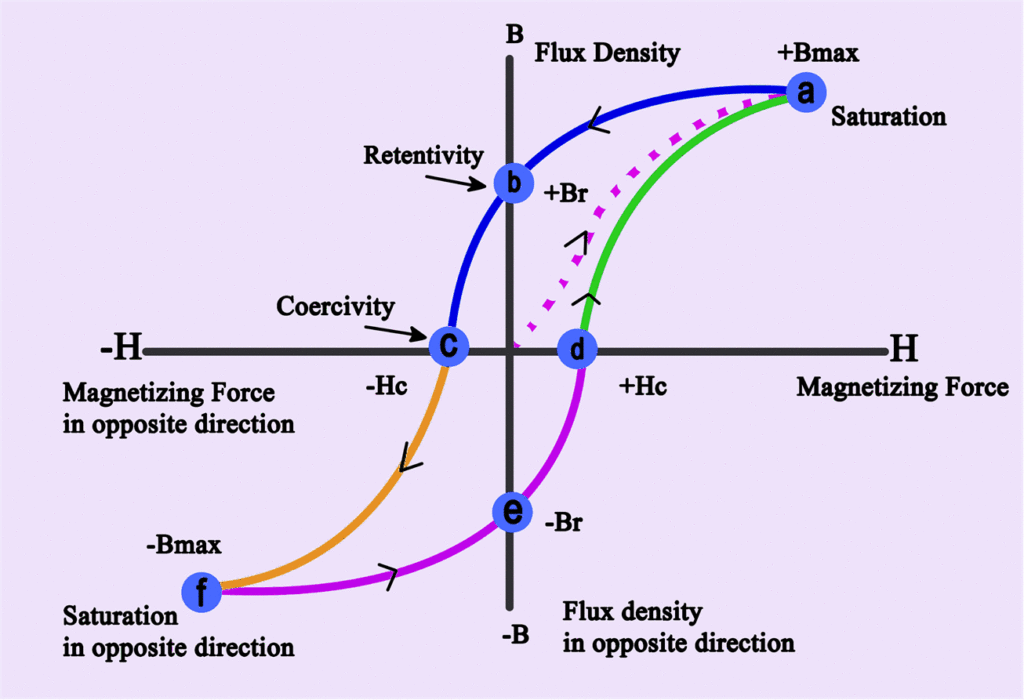Home / Magnetism / Hysteresis Loop | Magnetization Curve

# Hysteresis Loop | Magnetization Curve

Want create site? Find Free WordPress Themes and plugins.

## Hysteresis Loop Definition

A curve, or loop, plotted on B-H coordinates showing how the magnetization of a ferromagnetic material varies when subjected to a periodically reversing magnetic field, is known as Hysteresis Loop.

Hysteresis Definition

Hysteresis is the lagging of the magnetization of a ferromagnetic material behind the magnetizing force H.

By using a graph having B-H coordinates, we can plot the hysteresis characteristics of a given ferromagnetic material. Such a curve is plotted in the following figure and called a hysteresis loop. By periodically reversing a magnetizing force, we can plot the changing values of B within the material.Actually, a hysteresis loop is a B-H curve under the influence of an AC magnetizing force. Values of flux density B are shown on the vertical axis and are in Tesla. Magnetizing force H is plotted on the horizontal axis.

In above figure, the specimen is assumed to be unmagnified, and the current is starting from zero in the center of the graph. As H increases positively, B follows the red dotted curve from origin to saturation point a, indicated by Bmax.

As H decreases to zero, the flux follows the curve ab and drops to Br which indicates the retentively or residual induction. This point represents the amount of flux remaining in the core after the magnetizing force is removed.

When H starts in the negative direction, the core will lose its magnetism, as shown by following the curve from point b to c. The amount of magnetizing force required to completely demagnetize the core is called the coercive force and is designated as –Hc in the figure.

As the peak of the negative cycle is approached, the flux follows the portion of the curve labeled cd. Point –Bmax represents saturation in the opposite direction from Bmax . From point d, the – H value decreases to point e, which corresponds to a zero magnetizing force. Flux –Br still remains in the core.

A coercive force of +Hc is required to reduce the core magnetization to zero. As the magnetic force continues to increase in the positive direction, the portion of the loop from point f to a is completed. The periodic reversal of the magnetizing force causes the core flux to repeatedly trace out the hysteresis loop.

## Demagnetization or Degaussing

The process by which the magnetization within the ferromagnetic materials is reduced to zero by exposing it to a strong alternating magnetic field that is gradually reduced to zero.

To demagnetize any magnetic material, we must reduce its residual magnetism Br to zero. This can be done by connecting a suitable coil to a source of alternating current and placing it close to the object to be degaussed. Slowly moving the coil and object away from each other, causes the hysteresis loop to become progressively small. Finally, a point is reached where the loop is reduced to zero and no residual magnetism remains.

From the hysteresis loop, we can conclude different magnetic properties of a material such as:

Reluctance– The opposition that a magnetic circuit presents to the passage of magnetic lines through it.

Retentivity– The ability of a ferromagnetic material to retain residual magnetism is termed its retentivity.

Residual Magnetism– The magnetism remaining after the external magnetizing force is removed.

Coercive Force – The magnetic field strength required to reduce the residual magnetism to zero is termed the coercive force.

Permeability– Permeability is the measure of the ease, with which magnetic lines of force pass through a given material.

Did you find apk for android? You can find new Free Android Games and apps.

### About Ahmad FaizanMr. Ahmed Faizan Sheikh, M.Sc. (USA), Research Fellow (USA), a member of IEEE & CIGRE, is a Fulbright Alumnus and earned his Master’s Degree in Electrical and Power Engineering from Kansas State University, USA.